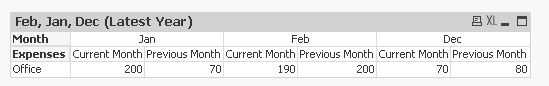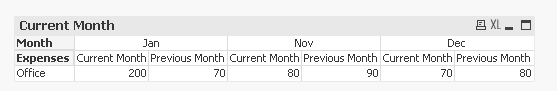# QlikView App Dev

Discussion Board for collaboration related to QlikView App Development.

Announcements
Our next Qlik Insider session will cover new key capabilities. Join us August 11th REGISTER TODAY
cancel
Showing results for
Did you mean:Partner

## Current vs Previous Month with month as a dimension..but it should be for Jan, Dec, Nov(Current month vs previous two months)

Hi,

I want to show Current month Sales and previous month sales with the month as a dimension..for 3 months (Current month & previous 2 months)

Its Urgent...

I achieved previous month vs current month, but how can I restrict for 3 months only.

Regards,

kavita

1 Solution

Accepted SolutionsMVP

Changed the expression for previous month:

=If(Sum({<Date = {"\$(='>='&Date(AddMonths(Max(Date), -2), 'D/M/YYYY')&'<='&Date(Max(Date), 'D/M/YYYY'))"}>}Act_Amt) > 0,

If(Num(Month) > 1, Before(Sum({<Month, [Month Year], Date = {"\$(='>='&Date(AddMonths(Max(Date), -3), 'D/M/YYYY')&'<='&Date(Max(Date), 'D/M/YYYY'))"}, Year>}Act_Amt)),

After(Sum({<Month, [Month Year], Date = {"\$(='>='&Date(AddMonths(Max(Date), -3), 'D/M/YYYY')&'<='&Date(Max(Date), 'D/M/YYYY'))"}, Year>}Act_Amt), 11)))14 RepliesMVP

Try these two expressions:

2)

If(Num(Month) > 1, Before(Sum({<Month, [Month Year], Date, Year>}Act_Amt)), After(Sum({<Month, [Month Year], Date, Year>}Act_Amt), 11)))MVP

You mentioned seeing Jan, Dec and Nov data. The max date in your attached database is Feb 2014. You don't want to see Feb data? and do you want to see all years of data or just data for the most recent year?MVP

I case you want to see all years, you can try these expressions:

1) =Sum({<Month = {\$(=Concat({<Date = {"\$(='>='&Date(AddMonths(Max(Date), -3), 'D/M/YYYY')&'<='&Date(AddMonths(Max(Date), -1), 'D/M/YYYY'))"}>} Chr(39) & Month & Chr(39), ','))}>}Act_Amt)

2)

=If(Sum({<Month = {\$(=Concat({<Date = {"\$(='>='&Date(AddMonths(Max(Date), -3), 'D/M/YYYY')&'<='&Date(AddMonths(Max(Date), -1), 'D/M/YYYY'))"}>} Chr(39) & Month & Chr(39), ','))}>}Act_Amt) > 0,

If(Num(Month) > 1, Before(Sum({<Month, [Month Year], Date, Year>}Act_Amt)), After(Sum({<Month, [Month Year], Date, Year>}Act_Amt), 11)))Partner
Author

Yess.. Feb 2014...MVP

Updated application attached.Partner
Author

In latest year table, Feb previous month should be Jan-200MVP

Changed the expression for previous month:

=If(Sum({<Date = {"\$(='>='&Date(AddMonths(Max(Date), -2), 'D/M/YYYY')&'<='&Date(Max(Date), 'D/M/YYYY'))"}>}Act_Amt) > 0,

If(Num(Month) > 1, Before(Sum({<Month, [Month Year], Date = {"\$(='>='&Date(AddMonths(Max(Date), -3), 'D/M/YYYY')&'<='&Date(Max(Date), 'D/M/YYYY'))"}, Year>}Act_Amt)),

After(Sum({<Month, [Month Year], Date = {"\$(='>='&Date(AddMonths(Max(Date), -3), 'D/M/YYYY')&'<='&Date(Max(Date), 'D/M/YYYY'))"}, Year>}Act_Amt), 11)))Partner
Author

Okay..il try n let u know..

I just want to confirm...If Feb comes. it will work ??

The output should be dynamic...as month comesMVP

Feb seems to be already there so you are already seeing it now. If March comes you will see March. It is dynamicCommunity Browser